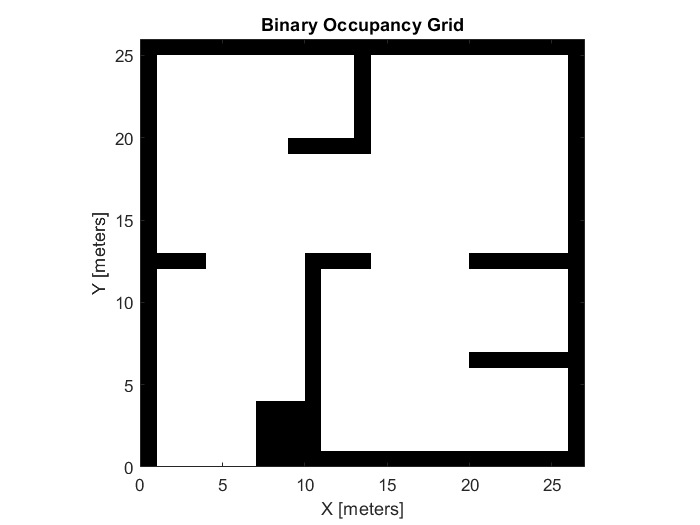# Mapping with Known Poses

This example shows how to create a map of an environment using range sensor readings and robot poses for a differential drive robot. You create a map from range sensor readings that are simulated using the `rangeSensor` object. The `differentialDriveKinematics` motion model simulates driving the robot around the room based on velocity commands. The `rangeSensor` gives range readings based on the pose of the robot as it follows the path.

### Reference Map and Figures

Load a set of example binary occupancy grids from `exampleMaps`, including `simpleMap`, which this example uses.

`load exampleMaps.mat`

Create the reference binary occupancy map using `simpleMap` with a resolution of 1. Show the figure and save the handle of the figure.

```refMap = binaryOccupancyMap(simpleMap,1); refFigure = figure('Name','SimpleMap'); show(refMap);```Create an empty map of the same dimensions as the selected map with a resolution of 10. Show the figure and save the handle of the figure. Lock the axes at the size of the map.

```[mapdimx,mapdimy] = size(simpleMap); map = binaryOccupancyMap(mapdimy,mapdimx,10); mapFigure = figure('Name','Unknown Map'); show(map);```### Initialize Motion Model and Controller

Create a differential-drive kinematic motion model. The motion model represents the motion of the simulated differential-drive robot. This model takes left and right wheels speeds or linear and angular velocities for the robot heading. For this example, use the vehicle speed and heading rate for the `VehicleInputs`.

`diffDrive = differentialDriveKinematics("VehicleInputs","VehicleSpeedHeadingRate");`

Create a pure pursuit controller. This controller generates the velocity inputs for the simulated robot to follow a desired path. Set your desired linear velocity and maximum angular velocity, specified in meters per second and radians per second respectively.

`controller = controllerPurePursuit('DesiredLinearVelocity',2,'MaxAngularVelocity',3);`

### Set Up Range Sensor

Create a sensor with a max range of 10 meters. This sensor simulates range readings based on a given pose and map. The reference map is used with this range sensor to simulate collecting sensor readings in an unknown environment.

```sensor = rangeSensor; sensor.Range = [0,10];```

### Create the Planned Path

Create a path to drive through the map for gathering range sensor readings.

`path = [4 6; 6.5 12.5; 4 22; 12 14; 22 22; 16 12; 20 10; 14 6; 22 3];`

Plot the path on the reference map figure.

```figure(refFigure); hold on plot(path(:,1),path(:,2), 'o-'); hold off```

Set the path as the waypoints of the pure pursuit controller.

`controller.Waypoints = path;`

### Follow Path and Map Environment

Set the initial pose and final goal location based on the path. Create global variables for storing the current pose and an index for tracking the iterations.

```initPose = [path(1,1) path(1,2), pi/2]; goal = [path(end,1) path(end,2)]'; poses(:,1) = initPose';```

Use the provided helper function `exampleHelperDiffDriveCtrl`. The helper function contains the main loop for navigation the path, getting range readings, and mapping the environment.

The `exampleHelperDiffDriveControl` function has the following workflow:

• Scan the reference map using the range sensor and the current pose. This simulates normal range readings for driving in an unknown environment.

• Update the map with the range readings.

• Get control commands from pure pursuit controller to drive to next waypoint.

• Calculate derivative of robot motion based on control commands.

• Increment the robot pose based on the derivative.

You should see the robot driving around the empty map and filling in walls as the range sensor detects them.

`exampleHelperDiffDriveCtrl(diffDrive,controller,initPose,goal,refMap,map,refFigure,mapFigure,sensor)````Goal position reached ```

### Differential Drive Control Function

The `exampleHelperDiffDriveControl` function has the following workflow:

• Scan the reference map using the range sensor and the current pose. This simulates normal range readings for driving in an unknown environment.

• Update the map with the range readings.

• Get control commands from pure pursuit controller to drive to next waypoint.

• Calculate derivative of robot motion based on control commands.

• Increment the robot pose based on the derivative.

```function exampleHelperDiffDriveControl(diffDrive,ppControl,initPose,goal,map1,map2,fig1,fig2,lidar) sampleTime = 0.05; % Sample time [s] t = 0:sampleTime:100; % Time array poses = zeros(3,numel(t)); % Pose matrix poses(:,1) = initPose'; % Set iteration rate r = rateControl(1/sampleTime); % Get the axes from the figures ax1 = fig1.CurrentAxes; ax2 = fig2.CurrentAxes; for idx = 1:numel(t) position = poses(:,idx)'; currPose = position(1:2); % End if pathfollowing is vehicle has reached goal position within tolerance of 0.2m dist = norm(goal'-currPose); if (dist < .2) disp("Goal position reached") break; end % Update map by taking sensor measurements figure(2) [ranges, angles] = lidar(position, map1); scan = lidarScan(ranges,angles); validScan = removeInvalidData(scan,'RangeLimits',[0,lidar.Range(2)]); insertRay(map2,position,validScan,lidar.Range(2)); show(map2); % Run the Pure Pursuit controller and convert output to wheel speeds [vRef,wRef] = ppControl(poses(:,idx)); % Perform forward discrete integration step vel = derivative(diffDrive, poses(:,idx), [vRef wRef]); poses(:,idx+1) = poses(:,idx) + vel*sampleTime; % Update visualization plotTrvec = [poses(1:2, idx+1); 0]; plotRot = axang2quat([0 0 1 poses(3, idx+1)]); % Delete image of the last robot to prevent displaying multiple robots if idx > 1 items = get(ax1, 'Children'); delete(items(1)); end %plot robot onto known map plotTransforms(plotTrvec', plotRot, 'MeshFilePath', 'groundvehicle.stl', 'View', '2D', 'FrameSize', 1, 'Parent', ax1); %plot robot on new map plotTransforms(plotTrvec', plotRot, 'MeshFilePath', 'groundvehicle.stl', 'View', '2D', 'FrameSize', 1, 'Parent', ax2); % waiting to iterate at the proper rate waitfor(r); end end ```